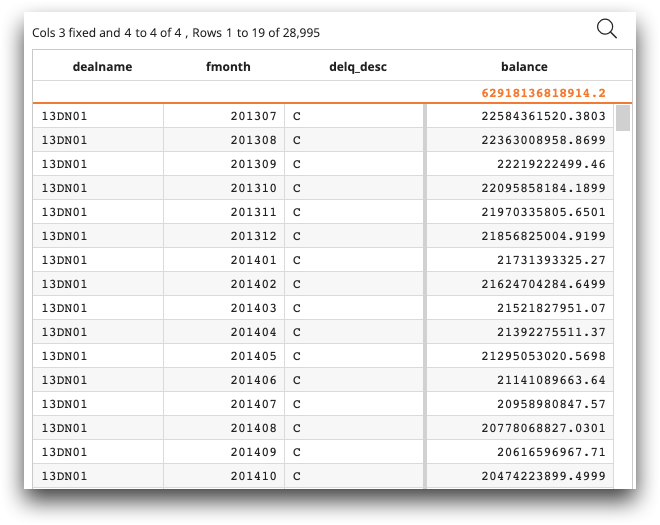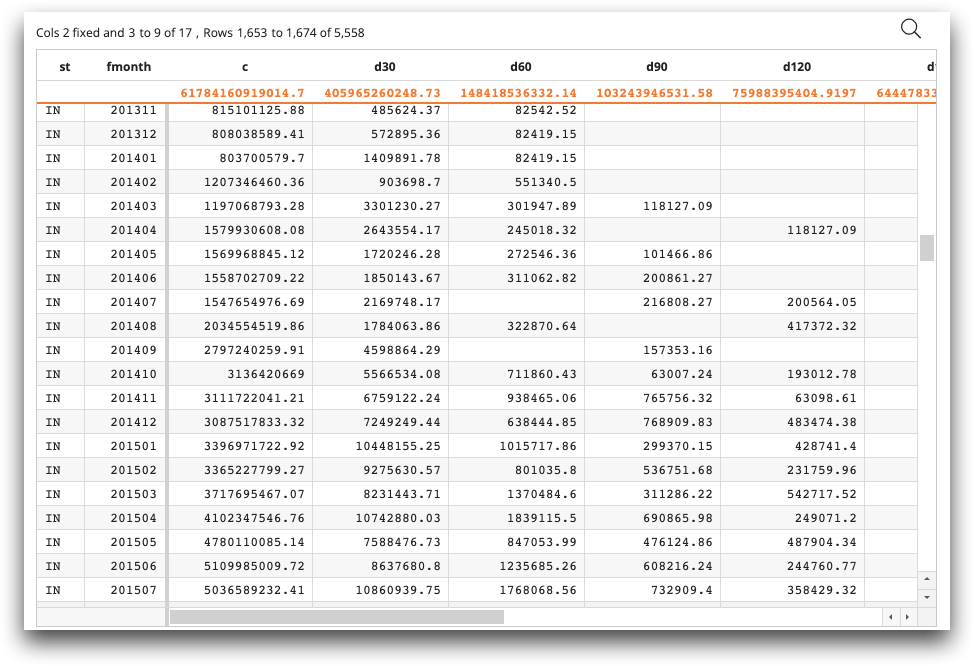# Using SQL in the 1010data Macro Language

You can get started writing SQL by opening Macro Language Workshop and writing SQL in the code editor.

You can select any 1010data table for ad hoc analysis in the SQL environment. The tag `<code language_="sql">` tells 1010data that you will be using SQL for your analysis. It is useful to enclose SQL syntax in ```<![CDATA[ ... ]]>``` to preserve white space formatting and to prevent text from being interpreted as XML. Finally, you can invoke the table with the SQL `SELECT` statement, enclosing its full 1010data pathname in double quotes.

The following is an example of selecting certain data in the `salesdetail` table for ad hoc analysis in the SQL environment:
```<code language_="sql">
<![CDATA[
SELECT * FROM "pub.doc.retail.salesdetail" WHERE "Store" IN (3,15)
]]>
</code>```

There are some basic guidelines for performing ad hoc queries in the SQL environment with `<code language_="sql">`:

• 1010data's SQL compliance allows users to query their data through SELECT statements. Loading data through operations such as `INSERT INTO` or modifying the database through operation such as ```CREATE TABLE``` are not supported.
• 1010data uses both column names and column labels to name columns. The SQL engine, by default, refers to columns by their 1010data labels, not their 1010data names. You may prefer to use column names instead of column labels in your SQL code, since column names are usually shorter and contain no spaces. To use column names, use ```<code language_="sql" options_="{importwithlabels:0}">``` before your SQL code.
• The SQL engine requires double quotes around the 1010data pathname, so that the dots in the 1010data pathname are not interpreted as separate SQL catalogs/schemas/tables.

See `<code>` for a complete list of options for `<code language_="sql">`.

Another way to use SQL within 1010data is to add SQL schemas, tables, and column metadata explictly to the SQL environment. This allows you to map 1010data data types to SQL data types, as 1010data and SQL data types are different. The easiest way to add SQL metadata to your environment is through the SQL Metadata Tool within the 1010data Insights Platform. See SQL Metadata Tool within the 1010data Insights Platform User's Guide for more information.

You can also add SQL metadata programmatically, with operations in ```<do action_="sql">```, such as `<do action_="sql" add_="schema">`, ```<do action_="sql" add_="table">```, and `<do action_="sql" add_="column">`.

Once tables are added to the SQL environment and you have determined your SQL metadata, you can use SQL directly, without `<code language_="sql">`. Simply write your SQL code directly in Macro Language Workshop.

While it is possible to access and query 1010data tables solely with SQL, you can also combine SQL with 1010data Macro Language to get the most out of 1010data's platform. See Using a SQL/1010data hybrid for details.

## Example: Performing a SQL tabulation

The following example demonstrates how to add the table `pub.fin.risk_share.stacr.history` ad hoc to your environment with `<code language_="sql">`. `options_="{importwithlabels:0}">` tells the SQL engine to use the 1010data column names as SQL column names. The example then uses SQL to mimic the tabulation function in the 1010data Macro Language.
```<code language_="sql" options_="{importwithlabels:0}">
<![CDATA[
SELECT A.dealname,A.fmonth,A.delq_desc,SUM(A.act_endg_upb)
AS balance FROM (SELECT substr(id_loan,1,6) AS dealname,fmonth,act_endg_upb,
delq_sts,dt_zero_bal,payoff_reason,
CASE
WHEN delq_sts= 0 AND payoff_reason IS NULL THEN 'C'
WHEN delq_sts=1 AND payoff_reason IS NULL THEN '30'
WHEN delq_sts=2 AND payoff_reason IS NULL THEN '60'
WHEN delq_sts=3 AND payoff_reason IS NULL THEN '90'
WHEN delq_sts=4 AND payoff_reason IS NULL THEN '120'
WHEN delq_sts=5 AND payoff_reason IS NULL THEN '150'
WHEN delq_sts=6 AND payoff_reason IS NULL THEN '180+'
WHEN dt_zero_bal=fmonth AND payoff_reason=1 THEN 'VPP'
WHEN dt_zero_bal=fmonth AND payoff_reason=2 THEN 'TPS'
WHEN dt_zero_bal=fmonth AND payoff_reason=3 THEN 'SS'
WHEN dt_zero_bal=fmonth AND payoff_reason=4 THEN 'DIL'
WHEN dt_zero_bal=fmonth AND payoff_reason=8 THEN 'REO'
WHEN dt_zero_bal=fmonth AND payoff_reason=96 THEN 'DFCT'
WHEN dt_zero_bal=fmonth AND payoff_reason=97 THEN 'D180'
ELSE 'OTHER'
END
AS delq_desc FROM "pub.fin.risk_share.stacr.history")
AS A group by A.dealname,A.fmonth,A.delq_desc
]]>
</code>```## Example: Performing a SQL cross-tabulation

The following example demonstrates how you can mimic 1010data cross-tabulation functionality entirely within SQL code. The example shows the aggregated balances of different payment states for all loans in STACR.

In this example, the table `pub.fin.risk_share.stacr.history` was added to the SQL environment through the SQL Metadata Tool. Therefore, `<code language_="sql">` and `<![CDATA[...]]>` are not needed.

```SELECT C.st, C.fmonth,
SUM(CASE WHEN C.delq_desc='C' THEN C.act_endg_upb END) AS C,
SUM(CASE WHEN C.delq_desc='30' THEN C.act_endg_upb END) AS d30,
SUM(CASE WHEN C.delq_desc='60' THEN C.act_endg_upb END) AS d60,
SUM(CASE WHEN C.delq_desc='90' THEN C.act_endg_upb END) AS d90,
SUM(CASE WHEN C.delq_desc='120' THEN C.act_endg_upb END) AS d120,
SUM(CASE WHEN C.delq_desc='150' THEN C.act_endg_upb END)  AS d150,
SUM(CASE WHEN C.delq_desc='180+' THEN C.act_endg_upb END) AS d180p,
SUM(CASE WHEN C.delq_desc='VPP' THEN C.act_endg_upb END) AS VPP,
SUM(CASE WHEN C.delq_desc='TPS' THEN C.act_endg_upb END)  AS TPS,
SUM(CASE WHEN C.delq_desc='SS' THEN C.act_endg_upb END)  AS SS,
SUM(CASE WHEN C.delq_desc='DIL' THEN C.act_endg_upb END)  AS DIL,
SUM(CASE WHEN C.delq_desc='REO' THEN C.act_endg_upb END)  AS REO,
SUM(CASE WHEN C.delq_desc='DFCT' THEN C.act_endg_upb END)  AS DFCT,
SUM(CASE WHEN C.delq_desc='D180' THEN C.act_endg_upb END)  AS D180,
SUM(CASE WHEN C.delq_desc='OTHER' THEN C.act_endg_upb END) AS OTHER

FROM (SELECT st,fmonth,act_endg_upb,delq_sts,dt_zero_bal, payoff_reason,
CASE
WHEN delq_sts= 0 AND payoff_reason IS NULL THEN 'C'
WHEN delq_sts=1 AND payoff_reason IS NULL THEN '30'
WHEN delq_sts=2 AND payoff_reason IS NULL THEN '60'
WHEN delq_sts=3 AND payoff_reason IS NULL THEN '90'
WHEN delq_sts=4 AND payoff_reason IS NULL THEN '120'
WHEN delq_sts=5 AND payoff_reason IS NULL THEN '150'
WHEN delq_sts=6 AND payoff_reason IS NULL THEN '180+'
WHEN dt_zero_bal=fmonth AND payoff_reason=1 THEN'VPP'
WHEN dt_zero_bal=fmonth AND payoff_reason=2 THEN 'TPS'
WHEN dt_zero_bal=fmonth AND payoff_reason=3 THEN 'SS'
WHEN dt_zero_bal=fmonth AND payoff_reason=4 THEN 'DIL'
WHEN dt_zero_bal=fmonth AND payoff_reason=8 THEN 'REO'
WHEN dt_zero_bal=fmonth AND payoff_reason=96 THEN 'DFCT'
WHEN dt_zero_bal=fmonth AND payoff_reason=97 THEN 'D180'
ELSE 'OTHER'
END
AS delq_desc FROM "pub.fin.risk_share.stacr.history"
A INNER JOIN "pub.fin.risk_share.stacr.master" B ON A.id_loan=B.id_loan)
AS C GROUP BY C.st, C.fmonth order by C.fmonth,C.st```